# Python Scikit Learn: The Complete Guide

Scikit-learn is a machine learning library for Python. It has many features like regression, classification, and clustering algorithms, including SVMs, gradient boosting, k-means, random forests, and DBSCAN.

It is designed to work with Numpy and Pandas library. However, Scikit learn is written in Python (most of it), and some of its core algorithms are written in Cython(C extensions for Python) for even better performance.

Scikit-learn is used to build Machine Learning models. Using it for reading, manipulating, and summarizing data is not recommended as better frameworks like Pandas and NumPy.

## Python Scikit Learn

For this example, we will use two ways to run Scikit learn on your machine.

If you have successfully installed the virtual environment, please enter that folder and activate it using the following command.

`source bin/activate`

My virtualenv is started, and now I can list the packages I have installed on that environment using the following command.

`pip list`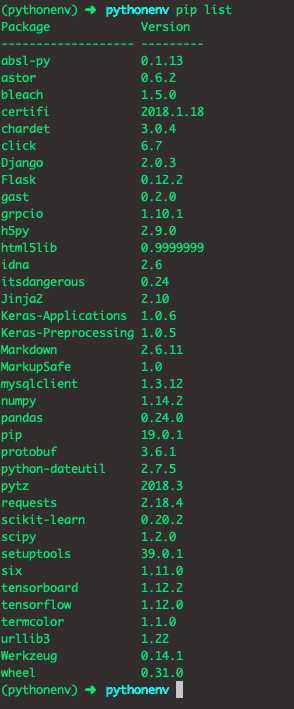I have already installed the Scikit Learn package.

You can install it using the following command.

`pip install scikit-learn`

If you are using Python Jupyter Notebook, you have already installed the Scikit learn package.

### Example

Now, you have two choices. If you want to use Jupyter Notebook, then you can use that, and if you are using virtualenv, write the code in a code editor like Visual Studio Code and run the file in the console.

For this example, I am using Python Jupyter Notebook. So, open up the notebook.

Let’s deep dive into the code and see the Scikit learn in action.

If we need to work with Scikit Learn, we must have some data.

Let’s create some data using NumPy

## Step 1: Import NumPy and Scikit learn

So, let’s import two libraries.

```import numpy as np
from sklearn.preprocessing import MinMaxScaler```

First, we imported the NumPy library, and then we imported the MinMaxScaler module from sklearn.preprocessing library.

MinMaxScaler module is used when we need to do feature scaling to the data.

Feature scaling means that in a particular column, you will find the highest value and divide all the values with that highest value. That means your column has only values between 0 and 1.

The MinMaxScaler is probably the most popular scaling algorithm working with large data sets, especially in building the Machine Learning model.

## Step 2: Create demo data using NumPy

Let’s create data using the NumPy library.

Write the following code in the next cell.

```demoData = np.random.randint(10, 100, (10 ,2))
demoData```

So, we have created random integer data between 10 to 100 with ten rows and two columns. Of course, the data is a random number generated, so that yours might be different. But focus on Sklearn algorithms.

See the output.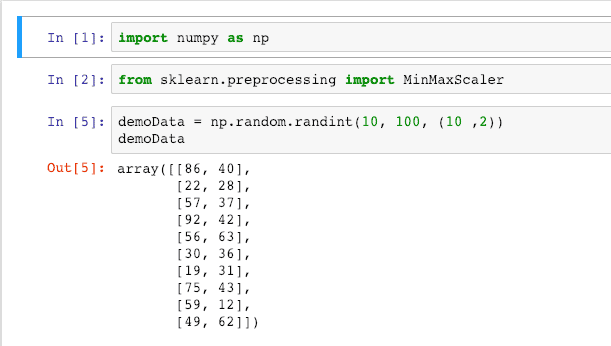## Step 3: Let’s transform data

As we have created demo data, now it is time to scale that data. So, we will use feature scaling.

First, create an object of MinMaxScalar.

Then pass the demoData to that MinMaxScalar’s fit_transform function.

```scalar_model = MinMaxScaler()
scalar_model.fit_transform(demoData)```

See the output.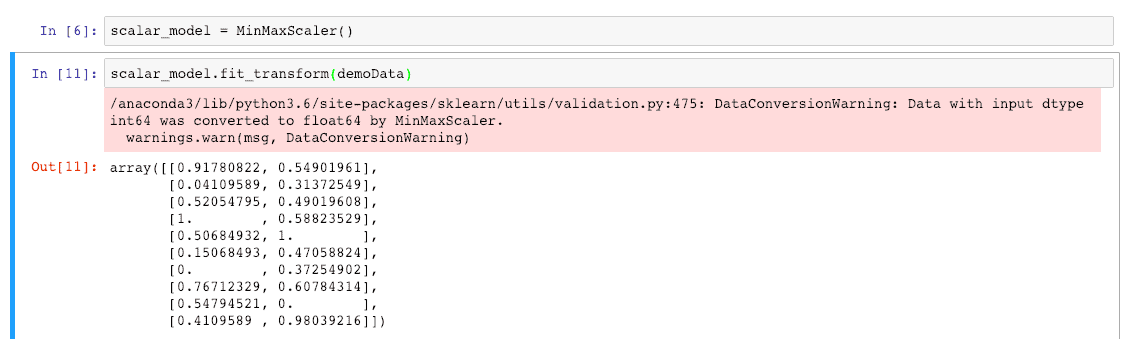Ignore the red warning; it tells us that we are converting integer data to floating data when we have transformed the value using MinMaxScalar.

## Step 4: Create a DataFrame

For splitting the data, first, we need to create a DataFrame, and for that, we need to import the Pandas library.

Now, we will create the demo data again, but this time, we will create a large dataset, create a DataFrame from that data, and then split that data to train and test.

Write the following code one by one cell.

```import numpy as np
from sklearn.preprocessing import MinMaxScaler```

Then write the following code in the next cell.

```demoData = np.random.randint(1, 500, (20 ,4))
demoData```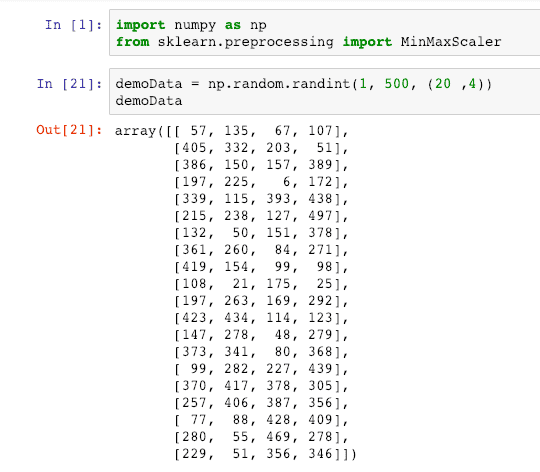Now transform the data to create feature scaling. So, write the following code inside the cell.

```scalar_model = MinMaxScaler()
feature_data = scalar_model.fit_transform(demoData)
feature_data```

It will display the scaled data.

See the output.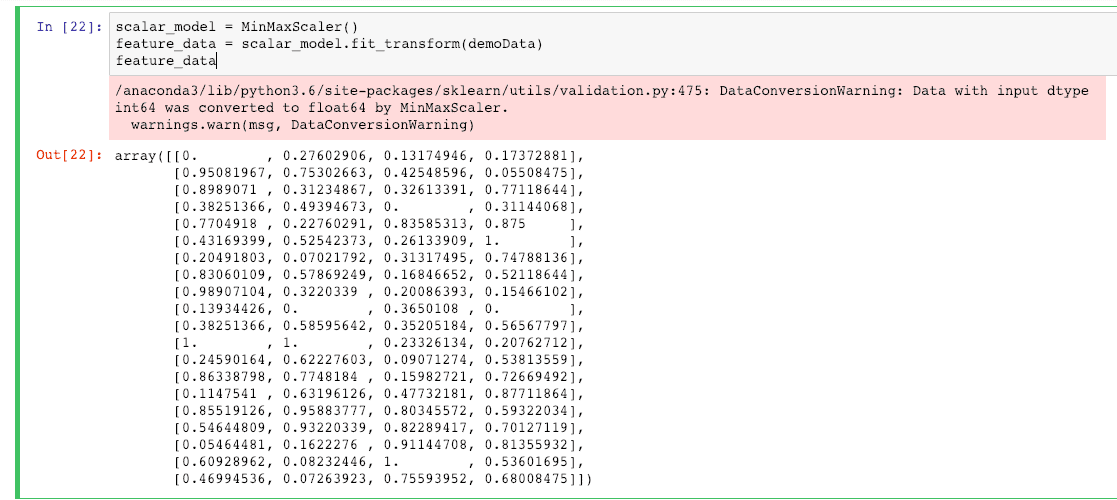The next step is to create a DataFrame from the above data.

So, write the following code in the next cell.

```import pandas as pd
df = pd.DataFrame(data=feature_data, columns=['k1', 'k2', 'k3', 'labels'])
df```

We have imported the pandas and created a DataFrame from the above feature_data. We have also defined the columns for the data. See the scaled data.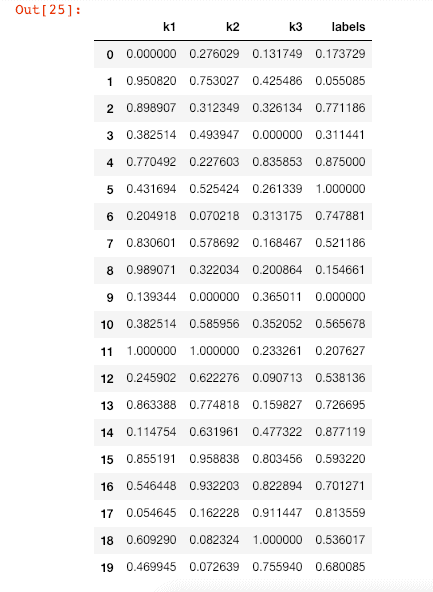We have three columns of featured data, and one column label is to predict the values. It is a supervised problem.

## Step 6: Split the data into Train and Test

First, let’s split the data between features and labels.

So, write the following code in the next cell.

```X = df[['k1', 'k2', 'k3']]
y = df['labels']```

Now, print the and y and see the output.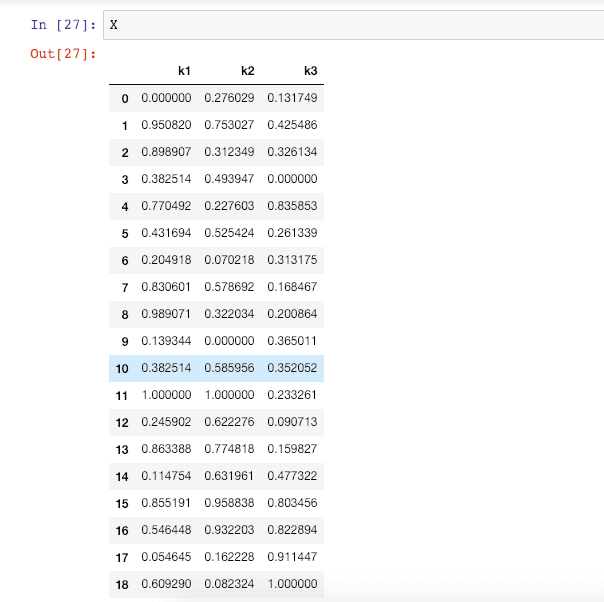See the in the output.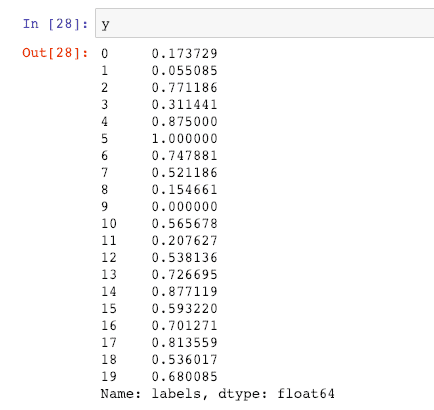So, now we have feature X and predict the label of the data y.

Let’s do the train and test split.

`from sklearn.model_selection import train_test_split`

Now, write the following code in the next cell.

`X_train, X_test, y_train, y_test = train_test_split(X, y, test_size=0.30, random_state=42)`

Run the cell.

What the above code does is that it will split the data between X_train and X_test with the 1:3. This Means that train data gets 70%, and test data get 30% from the DataFrame.

You can change the percentage you want for the test and train data, but this ratio is the standard ratio to split the data between train and test.

We can check how much data we get for the train and test data.

Type the following code in the next cell.

`X_train.shape`

Now, see the data of Y_train. See the output.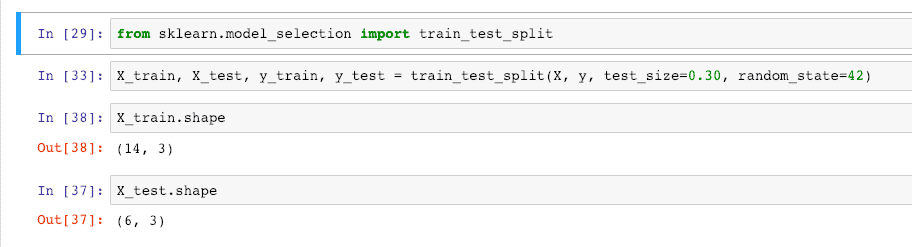You can see that X_train got the 14 rows, and Y_train got the six rows. So, it has split 70:30.

You can find more about the shape attribute here.

So, Scaling and splitting the dataset is the most crucial step in Machine Learning, and if you want to know how to prepare a dataset in Machine learning, check out this article.

That’s it.

### 3 thoughts on “Python Scikit Learn: The Complete Guide”

1.Hello krunal,

This is really great article and easy to understand. thanks for sharing.

keep up the good work.

2.3.